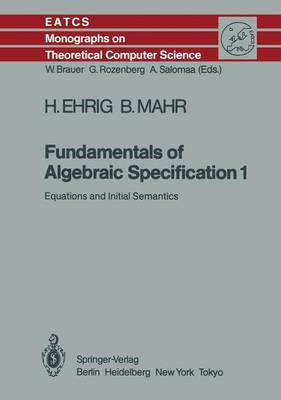•# Fundamentals of Algebraic Specification 1: Equations and Initial Semantics - Monographs in Theoretical Computer Science. An EATCS Series 6 (Paperback)

(author), (author)
£109.99
Paperback 321 Pages / Published: 23/11/2011
• We can order this

Usually dispatched within 3 weeks

The aim of this book is to present fundamentals of algebraic specifications with respect to the following three aspects: fundamentals in the sense of a carefully motivated introduction to algebraic specifications, which is easy to understand for computer scientists and mathematicians; fundamentals in the sense of mathematical theories which are the basis for precise definitions, constructions, results, and correctness proofs; and fundamentals in the sense of concepts, which are introduced on a conceptual level and formalized in mathematical terms. The book is equally suitableas a text book for graduate courses and as a reference for researchers and system developers.

Publisher: Springer-Verlag Berlin and Heidelberg GmbH & Co. KG
ISBN: 9783642699641
Number of pages: 321
Weight: 588 g
Dimensions: 244 x 170 x 18 mm
Edition: Softcover reprint of the original 1st ed. 198